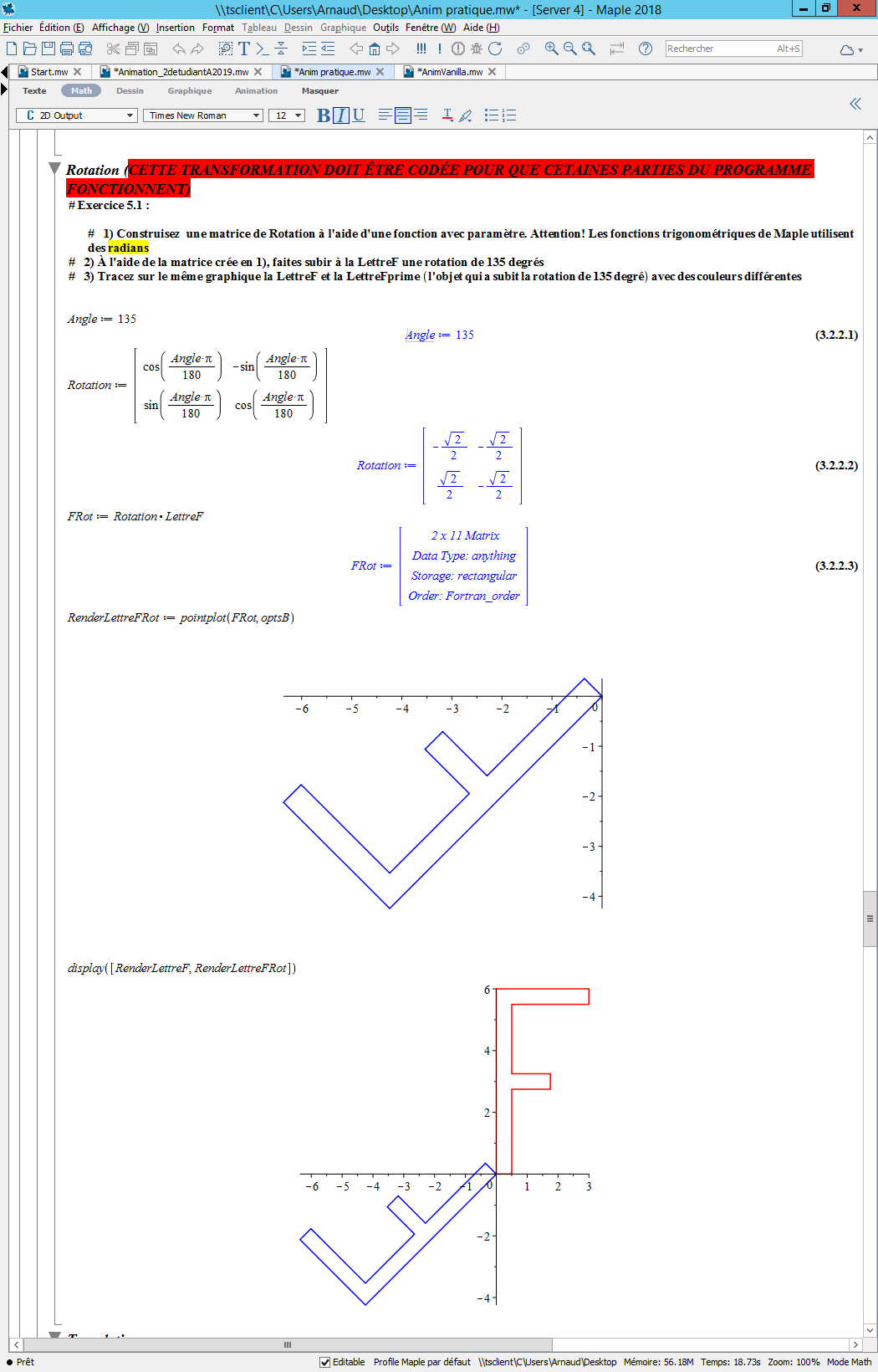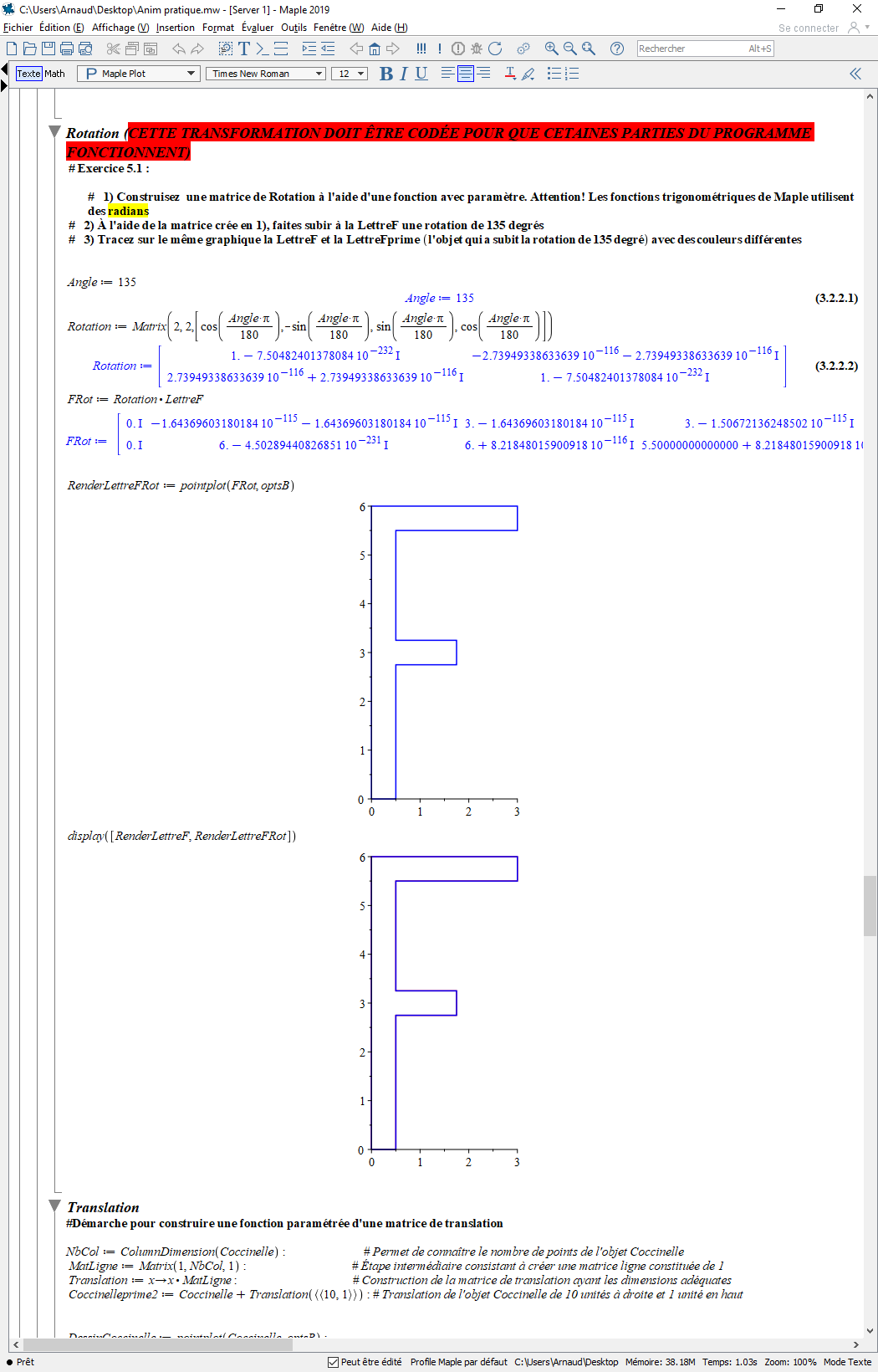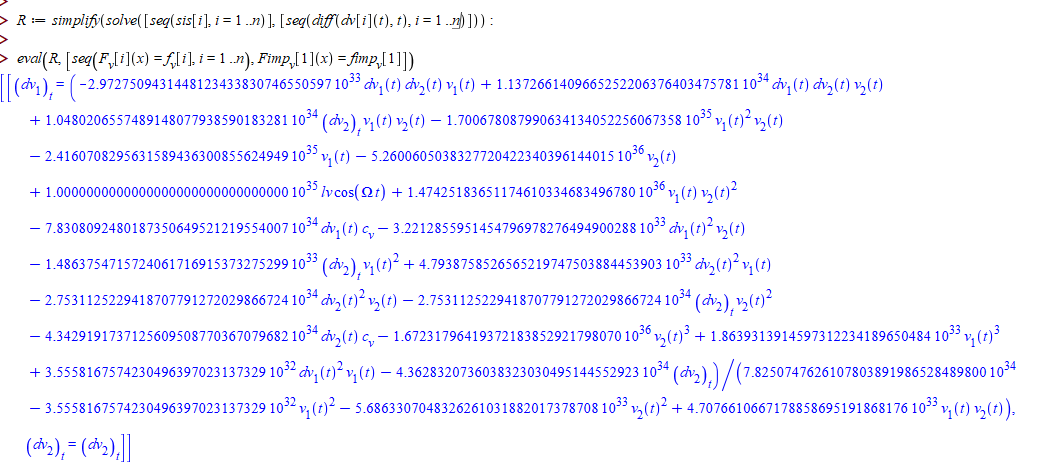## Which Bernstein has these result?...

Second terms coefficient = 2/3*x when (....)^2

9/16*x^2 when (...)^3

64/125*x^3 when (...)^4

625/1296*x^4

Which Bernstein has these result?

Which limit function of x^n = (n^(n-1))/((n+1)^(n-1))*x^(n-1)?

how to find and calculate?

Hi,

This is not an answer. I can't ask question, since I can't add any 'tags'! This problem appeared long time ago, but I hadn't solved it until now. So I ask here.

I have a question about the function coeffs(). I don't know why it cann't get coefficients for me.

question.mw

Thanks.

## Variable disappear after read file and create thre...

Variable disappear after read file and then pass parameter to create thread

## Are these bugs of maple 2019? ...

Hello people in mapleprimes,
I have a question.

I use maple2019 with mac os 10.14.6.

```\$ sw_vers
ProductName:	Mac OS X
ProductVersion:	10.14.6
BuildVersion:	18G95```

With maple2019, errors appears.

```> kernelopts(version);
Maple 2019.1, APPLE UNIVERSAL OSX, Jun 6 2019, Build ID 1403154

> assume(a>-1,b>0);
Originally a, renamed a~:
is assumed to be: FAIL

> assume(tau<1,tau>0,s<1,s>0):
> a_e1:=tau*s*(1+tau)<tau*s+tau+s-1:
> b_e2:=expand(lhs(a_e1)-rhs(a_e1))<0:
>  b_e3:=collect(b_e2,s,factor):
> solve(b_e2,s) assuming tau<1;
Error, (in assuming) when calling 'property/ConvertProperty'. Received: 'FAIL
is an invalid property'```

On the other hand, with maple2018, they do not.

```> kernelopts(version);
Maple 2018.2, APPLE UNIVERSAL OSX, Nov 16 2018, Build ID 1362973

> assume(a>-1,b>0);
Originally a, renamed a~:
is assumed to be: RealRange(Open(-1),1)

> assume(tau<1,tau>0,s<1,s>0):
> a_e1:=tau*s*(1+tau)<tau*s+tau+s-1:
> b_e2:=expand(lhs(a_e1)-rhs(a_e1))<0:
> b_e3:=collect(b_e2,s,factor):
> solve(b_e2,s) assuming tau<1;
1
[{-------- < s~}]
1 + tau~```

Are errors due to some bugs in maple2019 when being used with mac os 10.14.6?

Take care.

taro## How to do I make it work properly?...

Hi everybody!

Quick question for you guys. I have access to Maple 2019 on my main computer and Maple 2018 on a remote Desktop (but I don't want to use because it's super slow and bad (normal rdp stuff)). The problem I have is for the same document, same line, same instruction, Maple 2019 on my computer is failling to do it correctly (unable to do the matrix correctly) and in the Remote Maple 2018 it's doing it just fine. I really don't understand. If someone can give me a little help it would be highly apreciated!

Maple 2018 (what it's supposed to do):Maple 2019 (what it is not suppose to do):## How i can convert this mathematic code to maple...

How i convert the following mathematica code to Maple

f1[t^m_] := Gamma [m + 1] t^(m - a[t]) /Gamma[m + 1 + a[t]];
f1[t] := Gamma  t^(1 - a[t]) /Gamma[2 + a[t]];

f1[p_Plus] := Map[f1, p]
f1[c_*f_] := c*f1[f] /; FreeQ[c, t]
f1[c_] := 0 /; FreeQ[c, t]

f1[x^2*t^2 + t + x]

gives

t^(1 - a[t])/Gamma[2 + a[t]] + (2 t^(2 - a[t]) x^2)/Gamma[3 + a[t]]

## How to evaluate a table within a package module....

Glyph2.maple

The above link is a maple workbook containing the package Glyph2 together with the install script and two worksheets illustrating the difficulty I am having with the code in the procedure assignaltbasis (near the end of the code in Glyph2.mpl).

The problematic code is shown standalone is basisdemo worksheet, but reproduced below for reference. Interestingly, even though the table should be evaluated in a seq command, outside the module it works without eval. Inside the module evaluation does not make any difference.

indxs := [1, 2, 3, 4];
altbasis := [seq(seq(e[i[]], i = combinat:-choose(indxs, 2*k)), k = 1 .. 2)];
gen := table([seq(op(gen[i]) = bivec[i], i = 1 .. nops(gen))]);
exprs := [seq(exteriorp(seq(gen[op(2*i - 1 .. 2*i, b)], i = 1 .. nops(b)/2)), b = altbasis)];

The worksheet Basischangedemo shows how the exported procedure “assignaltbasis” is used in the module and the error that results if a bivector basis is used. From experimentation I have found that the error results because “assignaltbasis” passes the table name to the procedure “exteriorp” instead of the bivectors that should have resulted from evaluation of the table. Including the eval command within the seq procedure does not make any difference to the result. I am unclear whether this is a problem with evaluation of the table or a problem that results from namespace conflict.

I have a further namespace problem with the local variables, AltRoot, AltToStd, and StdToAlt which store the results from assignaltbasis.  If I declare these variables as exports, I get an error message “attempt to assign to a protected variable”. Therefore I added an exported procedure “ShowAltBasis” to display the results.  As you can see, this procedure produces the fully-qualified names of each blade making the result difficult to read. I would prefer to get rid of the “Glyph2:-” qualification.

The waters are muddied even further because the other worksheet, “basisdemo”, contains the core code extracted from assignaltbasis showing that the code using the table actually works (outside the module). From this I conclude that a namespace conflict exists within the module, preventing the table from fully evaluating.

My understanding is that within the module local and exported names are more or less equivalent, so I am puzzled why local and exported names in assignaltbasis are so problematic.

I would appreciate any advice about these issues. Clearly my understanding of maple’s namespaces is incomplete.

## How to plot y=0?...

Plot([x^2, subs(x=0, 2*x)], axis=[color=white]);

can not find the y=0 this red line

how to plot y=x^2 and x = 0 on the same graph to show the slope at x=0 ?

## How to derive to limit definition?...

How to derive from n*x^(n-1) to limit definition ?

## Output issue with solve command...

I am trying to solve a simple two-equation linear system with solve, but I keep getting this weird result where the magnitude order of the numerator and denominator don't cancel out. Whenever I have a linear system with floating-point numbers, more unknowns than equations and try to solve it for a specific set of variables, this happens.Any suggestions on how to get around this? Or do I need to solve it manually?

## How to proof exp(x) is transcendental function wit...

How to proof exp(x) is transcendental function without using calculus?

## How to write this equation in maple?...

How to write this equation in maple?

g(k-r-1);

conditions:=

1.r from 0 to k,

2.g(0)=1;

3.g(n)=0, where n not equal to 0.

## How to convert ODE to PDE?...

How to convert diff(f(t),t) + diff(g(t),t)

to diff(t(f,g), f)+ diff(t(f,g), g)

?